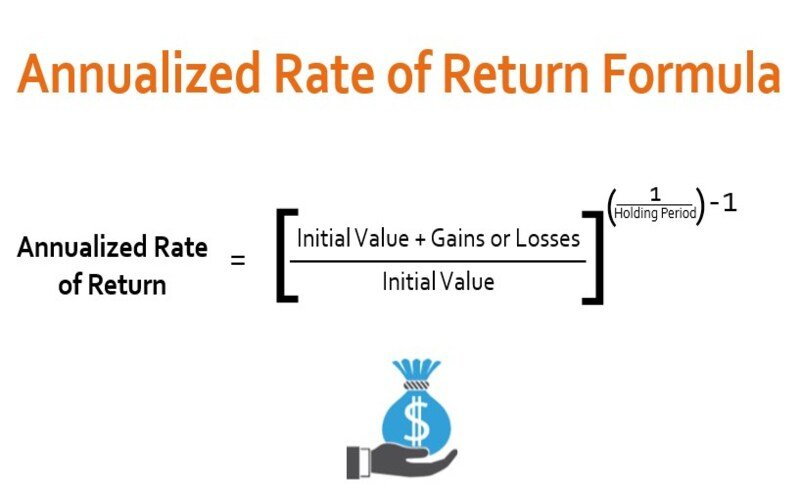Connect with us

Hi, what are you looking for?

DOGE0.070.84%SOL19.370.72%USDC1.000.01%BNB287.900.44%AVAX15.990.06%XLM0.080.37%

# Annualized Rate of Return: Definition, Examples, How To CalculateFile Photo: Annualized Rate of Return File Photo: Annualized Rate of Return

Annualized Rate of Return: Definition, Examples, How To Calculate

The equivalent yearly return that an investment would have received during a specific time is used to determine the annualized rate of return. Per the Global Investment Performance Standards, the returns of portfolios or composites for periods of less than a year may not be annualized.

This prohibits the “projected” performance for the remaining part of the year.

Knowledge of Annualized Rate

The 12-month timeframe is scaled down for annualized returns. Thanks to this scaling procedure, investors may objectively evaluate the returns of any assets over any period.

Calculation Using Annual Data

Calculating the annualized performance of an investment or index using yearly data uses the following data points:

P = principal, or initial investment

G = gains or losses

n = number of years

AP = annualized performance rate

The generalized formula, which is exponential to take into account compound interest over time, is:

AP = ((P + G) / P) ^ (1 / n) – 1

Examples of Annualized Rate of Return

Assume, for instance, that an investor puts \$50,000 into a mutual fund and that after four years, the investment is worth \$75,000, as shown. This represents a gain of \$25,000 over four years. The annualized performance is as follows:

AP = ((\$50,000 + \$25,000) / \$50,000) ^ (1/4) – 1

The annualized performance in this instance is 10.67 percent.

A 50% return is a gain of \$25,000 on a \$50,000 investment over four years. It is incorrect to state that the annualized return is 12.5 percent or 50% divided by four because compound interest is not considered. When the 10.67 percent result is reversed to compound over four years, the outcome is as anticipated:

\$75,000 = \$50,000 x (1 + 10.67%) ^ 4

It’s crucial to distinguish between annualized performance and actual performance. The pace at which an investment increases annually throughout the period to determine the ultimate valuation is known as the annualized performance. In this case, \$50,000 would increase to \$75,000 after four years with an annual return of 10.67%. But this doesn’t indicate the real yearly returns over the four years. \$50,000 becomes around \$75,000 after returns of 4.5 percent, 13.1 percent, 18.95 percent, and 6.7 percent. The same outcome is obtained with returns of 15%, -7.5%, 28.%, and 10.2%.

Utilizing Days for Calculation
The most accurate annualized return computation is based on industry norms for most investments and employs days rather than years. The exponent is the only difference in the formula:

AP = (1 – (365 / n) ((P + G) / P)

Assume the fund earned \$25,000 during 1,275 days, as in the prior example. Hence, the annualized return is:

AP = ((\$50,000 + \$25,000) / \$50,000) ^ (365/1275) – 1

In this case, the annualized performance is 12.31%.

How do annualized rates of return and yearly performance differ from one another?

An investment’s steady annualized rate of return is stated as a percentage over the years that it generates returns. Compound interest is also considered in the annualized return. Annual performance, which can vary greatly from year to year, is a snapshot of an investment’s profits and losses in a single year.

How can you better understand your investments using the annualized rate of return?

Investors maintain various investment kinds for differing lengths of time. Investors who want to compare the performance of several assets can do so using the annualized rate of return.

What is an annualized rate of return’s limitations?

Although it is not a guarantee, annualized rate of return can be a helpful tool to assess your investment prospects. The annualized rate of return on an investment might alter due to market volatility and other external factors.

An annual average over a specific period is used to determine the return on investment using the annualized rate of return. Investors can use the annualized rate of return to contrast various assets over a certain period. Market circumstances impact an annualized rate of return, which can alter over time.

Conclusion

• The annualized rate of return displays annual investment returns.
• The annualized rate examines average returns every year, whereas the rate of return examines profits or losses on assets across various periods.
• Over the years that the investment has generated returns, the annualized rate of return has remained constant and is given as a percentage.
• It contrasts with an investment’s yearly performance, which might change greatly from year to year.